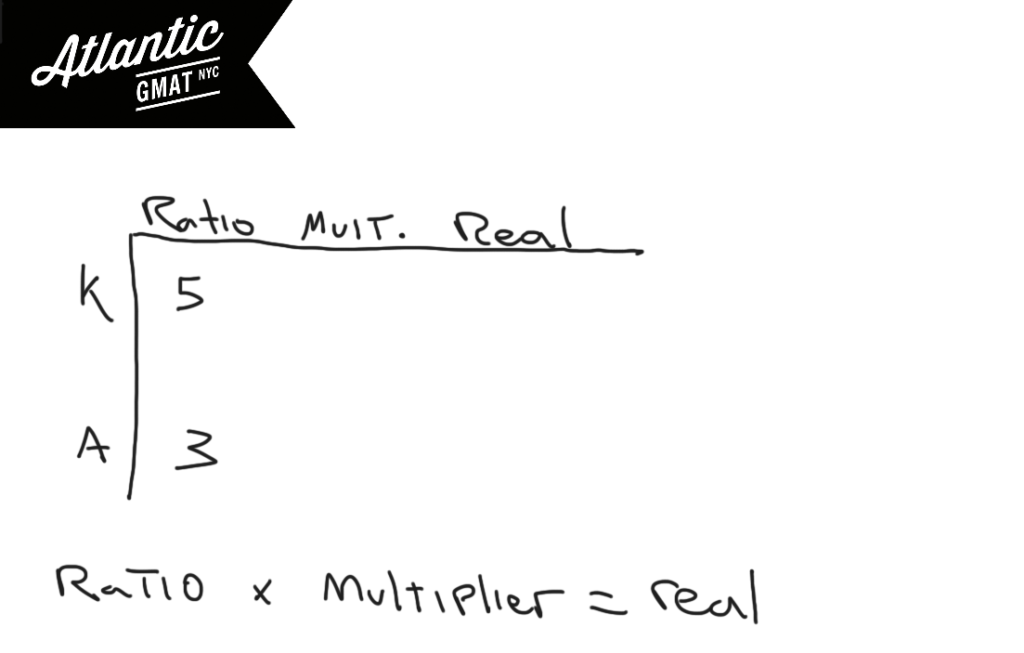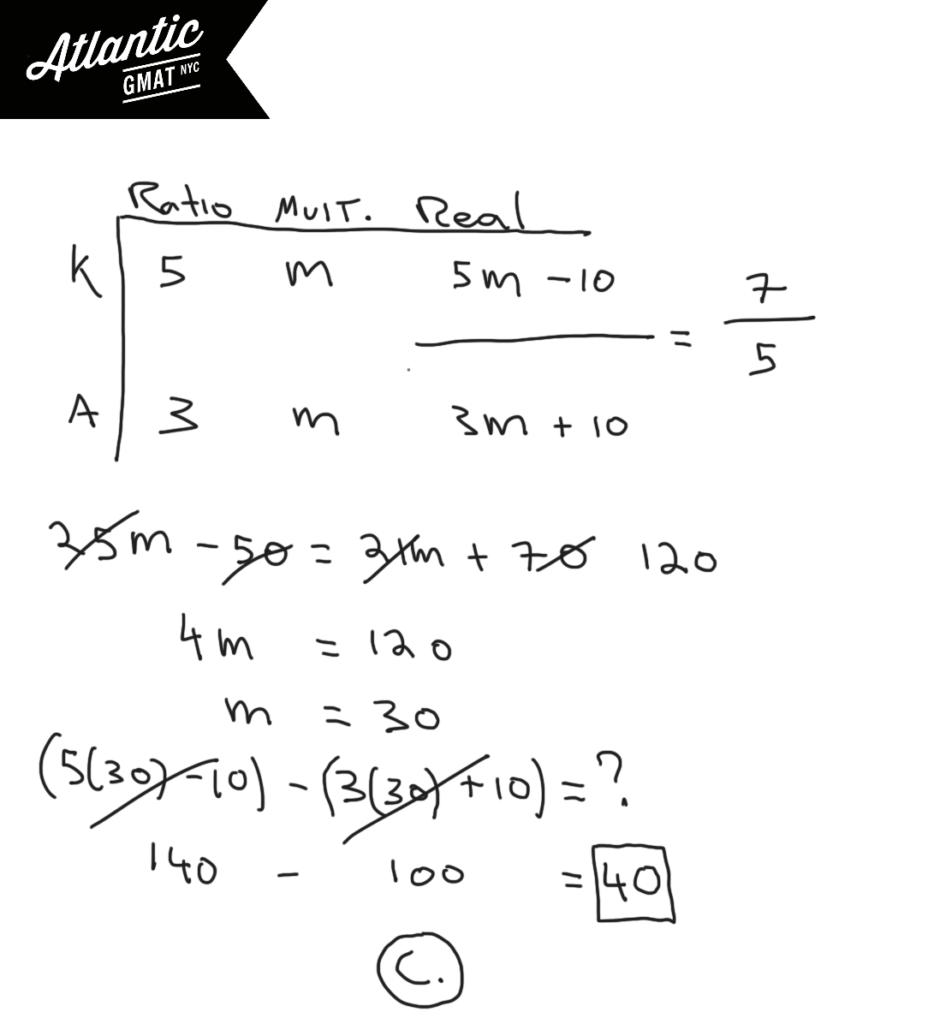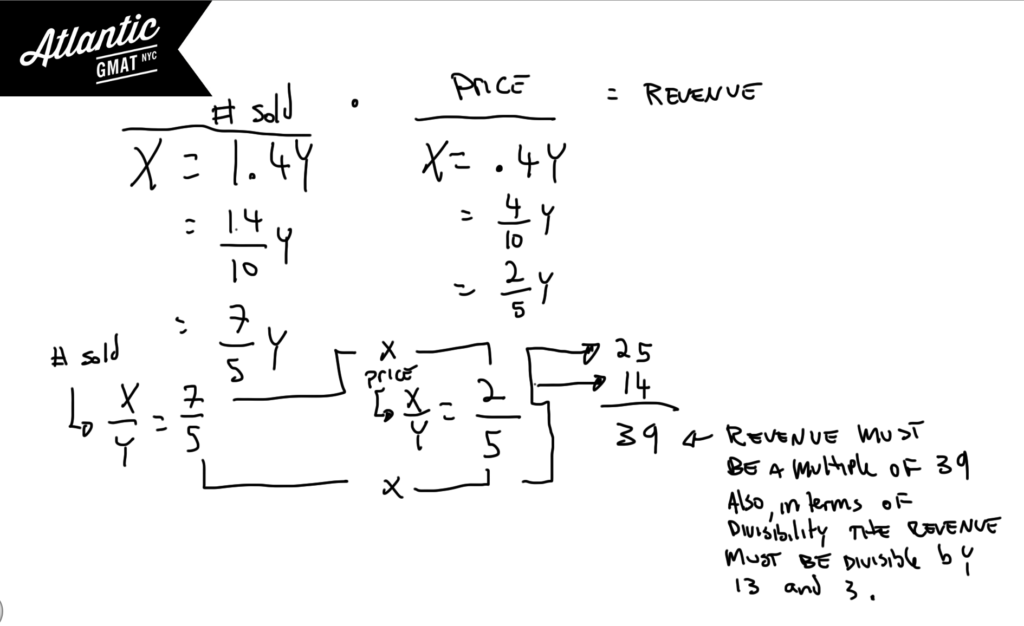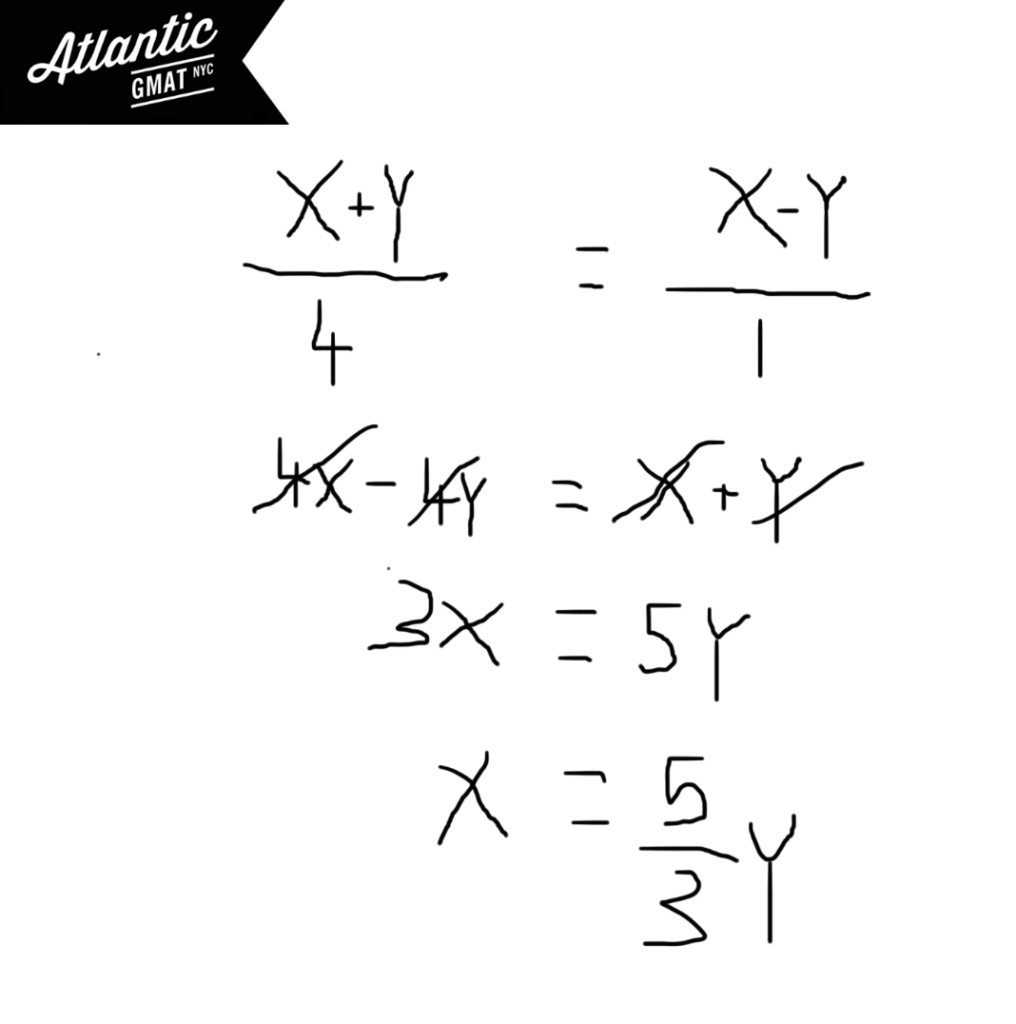Private GMAT Tutoring in NYC & Online

The number of stamps that Kaye and Alberto had were in the ratio of 5:3 respectively. After Kaye gave Alberto 10 of her stamps, the ration of the number of Kaye had to the number of Alberto had was 7:5. As a result of the gift, Kaye had how many more stamps than Alberto?

(A) 20
(B) 30
(C) 40
(D) 60
(E) 90

In GMAT tutoring we teach the "ratio box". It's nothing groundbreaking but helpful for organizing GMAT ratio information. The Kaye and Alberto stamp question is a very specific type of ratio question that comes up reasonably often. I call it a "new ratio" question because usually you're given a new ratio and then asked to solve for some of the real world values. Let's take what we know about Kaye and Alberto and hopefully make some inferences. Here's the ratio box with the information that we have:We don't know what the multiplier is so I'd always put in a variable. I usually use "M" for multiplier. Using variables that somewhat connect to what they represent is a good GMAT habit to get into. Then you can multiply across to get your real world values for the stamps that Kaye and Alberto have. To those you can add 10 stamps to Alberto and subtract 10 stamps from Kaye. After the stamp trading, the new ratio of stamps is 7:5. Now we can just solve for the "M" the multiplier and then solve for the difference of stamps (after the trade). Go ahead and comment with any questions or additions. Happy GMAT studies!# GMAT Question of the Day - Data Sufficiency - Inequalities

In a certain forest 1/5 of the trees are Walnuts and 1/6 of the trees are Birches. What is the number of birch trees in the forest?

(1) The difference between the number of Walnut trees and the number of Birch trees is greater than 10

(2) There are fewer than 350 total trees

# GMAT Question of the Day - Data Sufficiency - Geometry

A hardware store sells paint in two different containers. Container A is a cube with an edge of k. Container B is a right cylinder with a height of 5k. Assuming that each container is filled to capacity which container will cost less per unit of volume?

(1) Container A costs 1/3 as much as Container B

(2) Container B has an inside diameter which is less than 2k

# GMAT Question of the Day - Problem Solving - Ratio/Word Problem

On July 1st Company X and Company Y each sold their entire inventories of Widget Z. If Company X sold 40% more units of Widget Z than Company Y sold and charged 60% less than Company Y per unit of Widget Z, which of the following could be the combined revenue from the sale of Widget Z for Companies X and Y on July 1st?

A. 3200

B. 3900

C. 4200

D. 4800

E. 4900

## GMAT Question of the Day Solution

When a GMAT question asks for potential values (which of the following could be...) you probably need to think in terms of making a range (max/min) or in terms of ratios/divisibility. In this case we are most certainly dealing with proportions so think in terms of ratios. Ratios are the most basic relationship between values (You can almost think of them as the prime factorization of the relationship. Kind of.) In this GMAT question of the day we need to find the basic relationship between the two companies and then find a multiple of that relationship in the answer choices.# GMAT Question of the Day - Problem Solving - Word Problem/Ratio/Algebra

Alice and Carl each completed a portion of a certain job. Alice completed 20% of the job while Carl completed the rest. If they were each paid the same amount, what proportion of her payment must Alice give to Carl so that they each are paid the amount of the total that is proportionate with the amount of work that they each performed?

A. 1/2

B. 2/3

C. 3/5

D. 3/4

E. 5/8

## GMAT Question of the Day Solution

The GMAT Quant section has a whole bunch of word problems so it's important to feel comfortable with them. These questions tend to have a tough image but are often simple in concept (this question of the day has this feel). One thing that seems to help GMAT tutoring students: Taking time to carefully read the question. You do not need to solve the question as you read. You don't even have to take notes. The important thing is to process the information. To understand what the question is asking you to do. This is the time to make a broad plan for solving the question.

In this case Alice will need to give Carl part of her payment so that the pay per work is equal. Sounds like we can set up an equation with Carl on one side and Alice on the other. Pick a variable for the original payment (let's call it x) and pick a variable for the amount that Alice needs to lose from her side and that Carl will gain (let's call this y). Solve for y in terms of x and that will tell you the proportion of her payment that Alice needed to give to Carl.

You might be thinking: how do I deal with the different proportions of the job done by Alice and Carl? Well - look at it this way. We're dealing with 20% and 80% (.2 and .8). So the ratio is 1:4. Carl did 4 units of work to Alice's 1 unit of work. So Carl's money divided by his effort of 4 units must be equal to Alice's money divided by her effort of one unit.# CONTACT

Atlantic GMAT

405 East 51st St.

NY, NY 10022

(347) 669-3545

info@AtlanticGMAT.com